## Featured Posts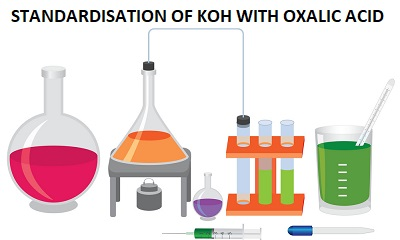## Standardization of KOH with Oxalic Acid

STANDARDISATION OF KOH (POTASSIUM HYDROXIDE) WITH OXALIC ACID

Determine the normality of the given solution of KOH using standard oxalic acid solution containing 6.2 gL-1

Principle:

The determination of normality is based on the reaction between KOH and oxalic acid as shown by the equation given below.

2KOH + H2C2O4  K2C2O4 + 2H2O

Here one mole of oxalic acid (two equivalents) reacts with two moles of KOH. Therefore, the equivalent mass of KOH is the same as its molecular mass (56).

Equivalent Mass of Oxalic acid = Molecular Mass/2 = 126/2 = 63

Equivalent Mass of KOH = Molecular Mass = 56

A known volume of KOH solution is titrated against the standard oxalic acid solution using phenolphthalein as the indicator. From the titre values, the normality and the mass per litre of KOH are calculated.

 Indicator End point In burette In pipette Phenolphthalein Pink to colourless Oxalic acid solution Potassium hydroxide solution

Procedure:

20 mL of the potassium hydroxide solution is pipetted out into a clean conical flask after rinsing the pipette with the same solution. One or two drops of phenolphthalein are added to it. It is then titrated against oxalic acid taken in the burette. The addition of acid is continued till the colour of the solution fades. The sides of the conical flask are washed with small quantities of water using a wash bottle. The titration is continued by adding acid in drops till the pink colour just disappears with a last single drop of acid. The end point is the disappearance of the pink colour. The titration is repeated to get concordant values. The results are tabulated as shown below. From the values, the normality and the mass per litre of KOH are calculated.

KOH x H2C2O4

 Expt. No: Volume of KOH Burette reading Volume of H2C2O4 Initial Final 1 2 3 20 20 20

Calculation

Volume of KOH solution, V1 = 20 mL

Let normality of KOH solution = N1

Normality of H2C2O4, N2 = Mass per litre/Eq. Mass = 6.2/63 = 0.0984

Let volume of H2C2O4 = V2 mL

N1 x V1 = N2 x V2

Normality of KOH, N1 = V2 x 0.0984/20

Mass per litre of KOH = N1 x 56g

Result

Normality of KOH = _____

Mass per litre of KOH = _____ g

NOTE:

Acidimetry: Acidimetry is the estimation of an alkali solution using a standard acid solution.

Alkalimetry: Alkalimetry is the estimation of an acid solution using a standard alkali solution. When an acid reacts with a base neutralization reaction takes place resulting in the formation of a salt and water. If both acid and base are strong then the resulting solution at the end point is neutral with pH = 7. On the other hand, if the acid or base is weak then the salt formed by the neutralization will undergo hydrolysis and the solution at the end point will be slightly alkaline or acidic. The indicators used in acidimetry and alkalimetry are phenolphthalein and methyl orange. These indicators are weak organic acids or bases. In acid-base titrations, acid should be taken in the burette and the base should be pippetted out into a conical flask.

A few other experiments in acidimetry and alkalimetry,

1. Determine the normality and the mass per litre of nitric acid using a standard solution of sodium hydroxide containing 4.1 gL-1.

2. Determine the strength and the mass per litre of sulphuric acid. You are provided with a standard solution of potassium hydroxide containing 5.5 gL-1.

3. Determine the normality and mass per litre of sodium carbonate. You are provided with hydrochloric acid solution containing 3.7 gL-1.

4. Determine the mass per litre of hydrochloric acid. You are supplied with a standard with a standard solution of potassium carbonate containing 7.0 gL-1.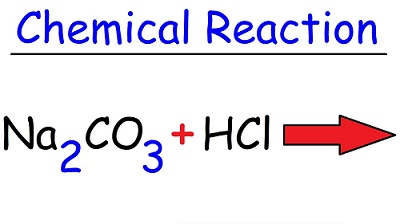## Standardization of Hydrochloric Acid using Sodium Carbonate

Standardization of Hydrochloric Acid using Sodium Carbonate

Determine the normality of hydrochloric acid using standard sodium carbonate solution containing 5.1 gL-1

Principle:

The determination of normality is based on the reaction between sodium carbonate and hydrochloric acid as follows.

Na2CO3 +2HCl => 2NaCl + H2O + CO2

It is clear from the equation that one mole of sodium carbonate (two equivalents) requires two moles of hydrochloric acid for complete reaction. Therefore, the equivalent mass of hydrochloric acid is same as its molecular mass.

Eq. mass of Na2CO3 = Molecular Mass/2 = 106/2 = 53

Eq. mass of HCl = Molecular Mass = 36.5

A known volume of standard sodium carbonate solution is titrated against the given hydrochloric acid using methyl orange as indicator. From the titre value, the normality and the mass per litre of hydrochloric acid are calculated.

 Indicator Methyl orange End point Yellow to pale orange red In burette HCl solution In pipette Na2CO3 solution

Procedure:

A clean burette is rinsed and then filled with the acid solution without air bubbles. A clean pipette is rinsed with the sodium carbonate solution. 20 mL of the standard sodium carbonate solution is then pipette out into a conical flask. One or two drops of methyl orange indicator are added to it to impart a golden yellow colour to the solution. It is then titrated against hydrochloric acid taken in the burette.

Wash the sides of the conical flask with water using a wash bottle near the end point. At the end point, the colour of the solution changes from golden yellow to pale orange red. The titration is repeated to get concordant values. The titre values are tabulated as shown below. From the values, the normality and the mass per litre of hydrochloric acid are calculated.

Na2CO3 x HCl

 Exp No: Volume of Na2CO3 Burette reading Volume of HCl used Initial Final 1 2 3 20 20 20

Calculation:

Volume of sodium carbonate solution, V1 = 20 mL

Normality of Na2CO3 solution, N1 = Mass per litre/Eq. Mass = 5.1/53 = 0.0962

Let volume of hydrochloric acid = V2 mL

Let normality of hydrochloric acid = N2

N1 x V1 = N2 x V2

Therefore, Normality of HCl, N2 = 0.0962 x 20/ V2

Mass per litre of HCl = N2 x 36.5 g

Result:

Normality of HCl = _______

Mass per litre of HCl = _______ g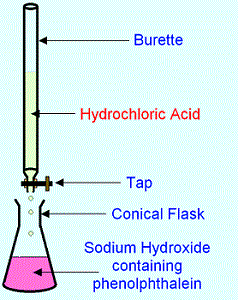## Standardization of Sodium Hydroxide with Hydrochloric Acid

STANDARDIZATION OF SODIUM HYDROXIDE WITH HYDROCHLORIC ACID

Determine the normality of the given sodium hydroxide solution using standard hydrochloric acid solution containing 3.6 gL-1

Principle

The determination of the normality of sodium hydroxide is based on the reaction between sodium hydroxide and hydrochloric acid as follows:

HCl + NaOH --> NaCl + H2O

From the equation it is clear that one mole of hydrochloric acid (one equivalent) requires one mole of sodium hydroxide for complete neutralisation. Therefore, the equivalent mass of sodium hydroxide is same as its molecular mass (40).

Eq. mass of HCl = M.Mass = 36.5

Eq. mass of NaOH = M.Mass = 40

A known volume of sodium hydroxide is titrated against the standard hydrochloric acid using phenolphthalein as indicator. Knowing the volume and the normality of the acid, the normality and the mass per litre of sodium hydroxide are calculated.

 Indicator End Point In burette In pipette Phenolphthalein Pink to colourless HCl solution NaOH solution

Procedure

A clean burette is rinsed with the standard hydrochloric acid solution. The burette is then filled with the solution, the stop cock is opened and the nozzle of the burette also is filled with the acid. After the air bubbles have escaped, the level of the acid is brought to the zero mark and the reading is noted. It is then clamped to a stand.

A pipette is rinsed with the sodium hydroxide solution. 20mL. of this solution is pipetted out into a clean conical flask. One or two drops of phenolphthalein are added to it. The conical flask is placed on a white porcelain tile below the burette. The level of nozzle tip of the burette should be inside the flask. It is then titrated against hydrochloric acid taken in the burette.

The acid is run into the sodium hydroxide solution in small quantities by regulating the stop-cock with the left hand. Mean while the flask is gently shaken with the right hand. The liquid in the conical flask is given a swirling motion. About one mL of the acid is added each time with shaking upto about 15 mL and then 0.5 mL each at a time till the colour of the solution fades. At this stage the sides of the conical flask are washed with small quantities of water using a wash bottle. There after the acid is added in drops. The titration is continued till the pink colour just disappears with a last single drop of acid. This is the end point. The final reading is noted. The difference between the final and initial readings gives the volume of acid added. The titration is repeated till concordant values are obtained. The titre values are tabulated as shown below. From the titre value, the normality and the mass per litre of sodium hydroxide are calculated.

NaOH x HCl

 Expt. No. Volume of NaOH Burette reading Volume of HCl Initial Final 1 2 3 20 20 20

Calculation

Volume of NaOH solution, V1 = 20 mL

Let normality of NaOH solution = N1

Normality of HCl, N2 = Mass per litre/Eq. Mass = 3.6/36.5

Let volume of HCl = V2 mL

N1 x V1 = N2 x V2

Therefore, Normality of NaOH, N1 = (V2 X N2)/V1

Therefore, Mass per litre of NaOH = Normality x Eq.mass = N1 x 40

Result

Normality of NaOH solution = ………..

Mass per litre of NaOH solution = ………g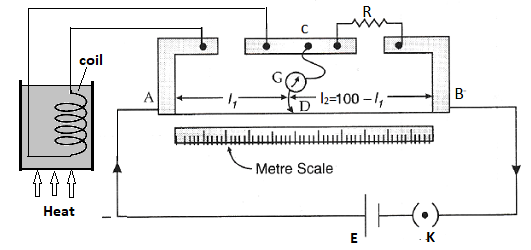## Temperature Dependence of Electric Resistance

Investigatory Project on Temperature Dependence of Electric Resistance

Aim: To investigate the dependence of the electric resistance of different materials (conductors and semiconductors) on different temperatures.

Apparatus: Metre bridge, A coil of copper wire (resistance about 10Ω), A semiconductor material (a carbon resistor of about 10Ω), A cell, Resistance box, Galvanometer etc.

Theory: The electrical resistance of a conductor is found to increase with increase of temperature (positive temperature coefficient of resistance), while that of a semiconductor decreases with increase of temperature (negative temperature coefficient of resistance).

Using metre bridge, the unknown resistance is calculated to be X = Rl/(100-l)

where R — Known resistance

l — Balancing length

Procedure:

i. Conductor: The coil of copper wire of about 10Ω resistance is placed inside a test tube containing liquid paraffin. The ends of the coil are connected to the tell gap of the metre bridge and the electrical connections are made as shown in the figure. Adjusting the position of jockey, the balancing point is determined. It is measured as 'l'. The temperature of the coil is noted using a thermometer. Similar way, balancing point is determined for different temperature (say 30, 50, 70, 90) by heating the liquid paraffin. A graph is plotted with temperature t along the X-axis and resistance X along the Y-axis. It is found to be a straight line with positive slope.

ii. Semiconductor: The experiment is performed with a given semiconductor, the resistance is determined for different temperatures. A graph is drawn with temperature t along the X-axis and resistance of semiconductor X along the Y-axis. It is found to be a straight line with negative slope.

Observations and Calculations

emf of the cell = …….. V

Range of resistance of box = 0 to ………

Range of thermometer scale = …… to ……

 Trail No: Material Temperature (t) Known resistance (R) Balancing length (l) (100 - l) Resistance, X = Rl/(100-l) - - °C ohm cm cm ohm 1 2 3 4 Conductor 1 2 3 4 Semi conductor

Temperature Resistance Graph

Report:

The resistance of both the conductor and the semiconductor are affected by the temperature changes.

i. Resistance of the conductor increases with increase of temperature.

ii Resistance of the semiconductor decreases with increase of temperature.## Zoology Viva Questions and Answers

Zoology Practical Viva Questions and Answers

Investigatory Project on Setting up and Maintenance of an Aquarium

1. What is an aquarium?

Aquarium is an open rectangular glass tank providing a condition similar to pond for the growth of fishes and water plants.

2. Name two ornamental fishes?

Gold Fish (Carassius auratus) and Guppy (Poecilia reticulata)

3. Name two water plants used in an aquarium?

Hydrilla and Valisnaria

Investigatory Project on Some insect pests and their control

4. What are pests?

Pests are organisms which destroy and damage crops and food grains during various stages of cultivation and storage.

5. Name any two paddy pests.

Leptocoriza acuta and Tryporyza incertulas.

6. Name a pest that attacks coconut palm.

Oryctes rhinoceros

Investigatory Project on Prepare an account of wild animals after visiting a Zoo or National Park

7. What is wildlife? Give example.

The uncultivated species of plants and animals living in their natural habitat is known as wild-life.

8. Give the scientific names of lion and elephant.

Panthera leo and Elephas maximus

9. What is Project Tiger?

It is a scheme to protect tigers from extinction. It was launched by the Govt. of India in 1973.

Investigatory Project on Control of Mosquitoes

10. Name the different species of mosquitoes found in your locality.

Anopheles, Culex and Aedes mosquitoes

11. Name two larvivorous fishes.

Gambusia and Aplocheilus

12. Name the diseases spread by Culex, Anopheles and Aedes

Culex – Elephantiasis

Anopheles – Malaria

Aedes – Dengue fever

Investigatory Project on Preparation of a report with illustration and photos from newspapers and magazines dealing with pollution

13. What is pollution?

Undesirable change in the physical, chemical and biological characteristic of air, water and land that may harmfully affect the living beings including man is called pollution.

14. What are pollutants?

The agents that cause pollution are known as pollutants.

15. Name any three air pollutants?

Carbon monoxide, Sulphur dioxide and Hydrocarbons

Eg: Domestic Sewage

Eg: DDT

Investigatory Project on DNA Model

17. What is DNA?

DNA or Deoxyribo Nucleic Acid is the genetic material in all organisms except certain viruses.

18. Who proposed the double helix model of DNA?

Watson and Crick (1953)

19. Name the nitrogen bases found in DNA.

20. Expand DNA

Deoxyribo Nucleic Acid

21. Which is the functional unit of DNA?

Gene

Investigatory Project on Survey of Human Phenotypic Characters

22. What is phenotype?

The visible characters of an organism are known as phenotypic characters.

23. What is genotype?

The genetic constitution of an organism is known as genotype.

24. What do you mean by hereditary characters?

The characters which transmit from parents to offspring are known as hereditary characters.

25. Name any two phenotypic characters that are heritable.

Fused ear lobes and rolling of tongue.

Investigatory Project on Preparation of Vermi Compost using household wastes, market wastes etc.

26. What is vermin compost?

It is the excretory product of earthworm rich in nutrients and minerals.

27. Name the elements found in vermin compost.

Nitrogen, potassium, and phosphorus

28. Mention the merits of vermin compost.

Vermi compost is safe, economic and ecofriendly.

Investigatory Project on Preparation of Rectal Ciliates in Amphibians

29. Name any two ciliates found in the rectum of frog.

Opalina and balantidium

30. What are ciliates?

Ciliates are unicellular animals. They possess cilia. They belong to the phylum protozoa.

Investigatory Project on Study on the Bird Fauna in the Locality

31. What is Ornithology?

Study of birds is known as ornithology

32. Who is called Bird Man of India?

Dr. Salim Ali

33. Name two birds found in your locality.

Crow and Bulbul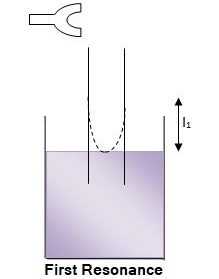## Vibrations of Air Column in Pipes

Investigatory Project – I

Vibrations of Air Column in Pipes – I

Aim: To study the factors affecting the resonant length of closed pipes.

Apparatus: Resonance column apparatus, Tuning forks, Rubber hammer, Metre scale etc.

Theory: In closed pipes, an antinode is formed at the open end and a node at the closed end. The distance between a node and the nearest antinode is λ/4. If ‘l’ is the length of the closed pipe, then l = λ/4.

Therefore, λ = 4l

The resonance occurs when the frequency of the tuning fork equals the frequency of natural vibrations of the closed pipe. Let v is the velocity of sound through air and n is the frequency of the tuning fork, then at resonance,

n = v/ λ = v/4l  or

l = v/4n

The velocity of sound is a constant at constant temperature.

Therefore, l 1/n or resonance length varies inversely with the frequency of the tuning fork.

Procedure:

Set the resonance column apparatus for experiment. Length of the air column is kept very small. A tuning fork of known frequency n, is excited and held horizontally near the mouth of the inner tube. The length of air column is measured. This is repeated again and the average length of the air column is determined. The experiment is repeated for tuning forks of different frequencies and the results are tabulated.

Observations and Calculations

Room temperature in the beginning = ____°C

Room temperature at the end = ____°C

 Frequency of the tuning fork (Hz) First resonating length Mean resonance length (cm) 1 (cm) 2 (cm)

Report:

At constant temperature the resonance length of closed pipes decreases with the increase in frequency of the driving source.

Investigatory Project – II

Vibrations of Air Column in Pipes – 2

Aim: To study the factors affecting the resonant length of closed pipe.

Apparatus: Resonance column apparatus, Tuning fork, Rubber hammer, Metre scale etc.

Theory: The wavelength (λ) corresponding to first resonating (l) is given by,

λ/4 = l +e, where end correction e = 0.3D, D is the inner diameter of the pipe.

The velocity of sound at room temperature (t) is v(t) = 4n(l+e) where n is the frequency of the tuning fork. At constant temperature, velocity of sound is a constant.

l 1/n

For constant n, λ is constant

Therefore, resonating length l (first resonating length observed) varies with diameter of the pipe.

Procedure:

Set the resonance column apparatus for experiment. Length of the air column is kept very small. A tuning fork of known frequency n, is excited and held horizontally over the mouth of the inner tube. The length of air column in the inner tube is slowly increased by raising the tube till a booming sound is heard. The length of the air column is measured. This is repeated again and the average length of the air column is determined. The experiment is repeated for pipes of different inner diameters.

Observations and Calculations

Frequency of the tuning fork, n = ___ Hz

Room temperature, t = ___°C

To study the vibration of resonating length with inner diameter of the pipe

 Trial No: Inner diameter of tube D (cm) First resonating length, l (cm) 1 2 3 4 5

Report:

The resonating length of closed pipe with its inner diameter at constant temperature is studied.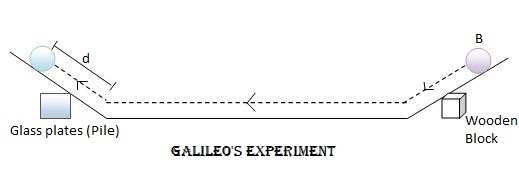## Galileo Experiment on Motion

Galileo Experiment on Motion Investigatory Project

Aim

To study the first law of motion by the double inclined track.

Apparatus

Double inclined track, Metal ball, Glass plate of same thickness, Wooden block etc. The double inclined track apparatus consists of a pair of inclined tracks, each of them can be independently set at a small angle of inclination.

Theory

A body will continue its state of rest or uniform motion along a straight line unless an external resultant force acts on it.

Procedure

Set up the double inclined track on the table. Clean the metal ball and the track using cotton or tissue paper. Insert the wooden block under the right arm at such a position that the track is inclined at a suitable angle (nearly 45°) with the horizontal. Then left arm is arranged at a suitable angle of inclination. This is done by placing a number of glass plates below it (say 14). A point 'B' is marked on the right arm which is at a distance 10 cm from the lower edge. The metal ball is released from 'B' and the distance 'd' to which it ascends in the left arm is noted. The experiment is repeated for different angle of inclinations in the left arm. This can be done by changing the number of glass plates below it. Since the glass plates are of same thickness, the slope of the left arm will be proportional to the number of glass plates (n).

A graph is plotted with number of glass plates (n) along the X-axis and the reciprocal of the distance 'd' along the Y-axis. The graph would be a straight line. On extrapolating the graph to slope zero (ie, n=0), it is found that 1/D is nearly zero or d = ∞, which implies that the body will continue its motion along a straight line. This verifies the validity of the first law of motion.

Observations and Calculations

 Trial No: of glass plates below the left arm (n) Distance of ascend along the left arm (d) 1/d 1 2 Mean (d) - - cm cm cm cm-1 1 2 3 4 5 6 14 12 10 8 6 4

Report

This experiment verifies that in the absence of an external force, a body in uniform motion would continue its motion indefinitely along a straight line.